Post

# More AI...(GEP) Gene Expression Programming in C# and .NET

• 16.3k
• 0
• 0

In the past we explored ways of using Genetic Algorithms (GA) to solve problems with a string of numbers that represent numbers.  For example, we used genetic algorithms in our MasterMind game as a computer player for solving the hidden color combination where each color was represented by an integer.  Gene Expression programming (GEP) is also a subset of Genetic Algorithms, except it uses genomes whose strings of numbers represent symbols.  The string of symbols can further represent equations, grammars, or logical mappings.  The genome can be mapped to a binary tree that you can walk along the nodes to evaluate the equation.  This is an extraordinarily powerful technique because now you are not mapping a GA to hard values, but to general symbols.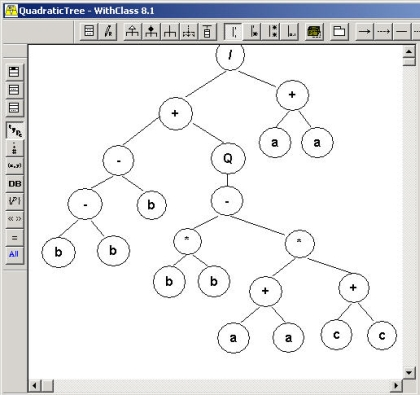Figure 1 - Quadratic Equation represented in a symbolic tree expression

Let's give an example of what we mean.  Lets let the value 0 represent the variable a,  1 represent multiplication, and 2 represent +.  So the string of numbers below:

0010021

Maps to the string

aa+aa**

Now how do you read this string as an equation?  Well actually that's completely up to your implementation.  In our implementation, we choose to read the equation in postfix order.  That means we walk the tree child, child, parent recursively.  Below is how the tree would look: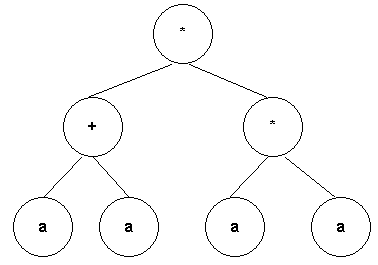Figure 2 - Expression Tree drawn using WithClass 2000 UML Tool

When written in InFix order (Child Parent Child), the symbolic order we are most familiar with, the resulting equation would look like the equation shown below:

(a+a) * (a*a)

Which is really  2a3  when you simplify the equation.

So what do we use this for?  Well,  as in all GA algorithms, we generate populations of genes and calculate fitness on those genes that depend upon the problem we want to solve.  Genes reproduce into the next generation of genes depending on how "fit" they are.  We can use Genetic Expressions for problems such as fitting points to a curve and coming up with the equation of the curve based on some sample values that fit on the curve.  When you plug the points into a genetic expression,  the symbolic expression that fits all the points the best have the highest fitness.

Instead of talking about theory,  let's give a practical example.  Let's use GEP to come up with the pythagorean theorem.   Pretend we don't yet know the pythagorean theorem, but we suspect there is a relationship between the sides of  right angle triangles and their corresponding hypotenuse.  Let's go out and measure a few triangles with really accurate rulers and come up with a set of lengths for each triangle (2 sides and a hypotenuse):

float[,] measure = new float[4,3]{{3,4,5}, {1, 1, 1.4142f}, {1, 2, 2.23607f}, {3, 1, 3.16228f}};

Now let's use our powerful GEP algorithm to come up with an equation mapping the sides of the triangle to the hypotenuse.  We'll pick the following symbols as symbols for our genome.

 0: a 1: b 2: * 3: / 4: + 5: - 6: Q (square root)

Granted, you might say, well how do we know to use square root and not hyperbolic tangent?  The truth is we could have added tangent, sine, cosine,  and perhaps we would get some interesting answers.  Even if we just used multiplication and addition, the GA would attempt to come up with the best set of symbols for the genome carrying the best fitness.  But clearly, having the right symbols in your symbol list for the solution will get you the best answer.

What sort of fitness function are we looking at for our Genome?  The fitness function should give us the highest number for results that occur when walking the genetic expression that are closest to the measured value of our hypotenuse.  This can be accomplished simply by taking the difference between the resulting value of plugging the sides of the measured triangle into the genetic expression minus the measured hypotenuse,  then taking the difference between a constant number (such as 100) and the value computed from the error.   Of course we don't want a fitness for only one of the measured values, so we then sum all of these fitnesses to produce a final fitness.  Below is the fitness function:

final fitness  =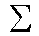(100 - |  geneticexpression(a, b) - hypotenuse |)

That should be enough to get us started in looking at our design and C# Code.  Below is the UML design of the GEP Algorithm.  If you've looked at my previous Genetic Algorithm article on C# Corner, this should probably look familiar to you.  The GA uses a Genome class which is part of a Population.  The EquationGenome is inherited from the Genome class to utilize all of its interfaces for performing the necessary tasks for a genetic algorithm such as mutation, reproduction, etc.  The EquationGenome contains the fitness function necessary to calculate the fitness of a symbolic gene.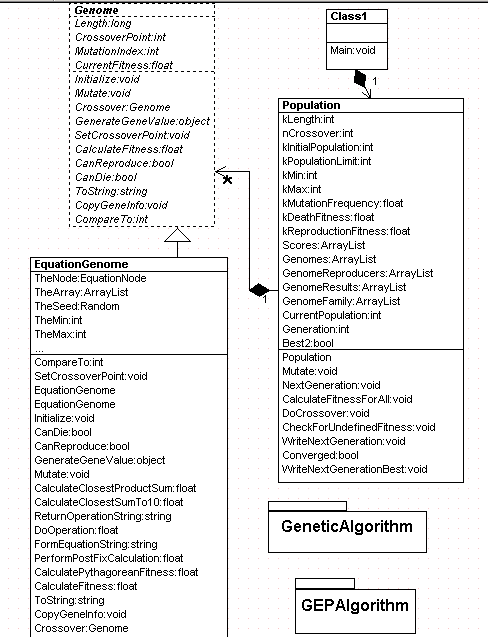Figure 3-GEP Algorithm UML Diagram Generated with WithClass 2000 UML Tool

Let's look at the fitness function for our EquationGenome.  It simply calls another function that calculates the fitness based on our sample measured triangles:

public override float CalculateFitness()
{
CurrentFitness = CalculatePythagoreanFitness(); // calculates the fitness for the triangle samples

return CurrentFitness;
}

Below is the code for CalculatePythagoreanFitness to calculate the full fitness of our symbolic function.  It loops through each of the set of measurements, and performs a postfix calculation on the symbolic string of our genome.  It then sums the error in these calculations together and subtracts each one from 100 (in order to produce a large fitness, you have to subtract a small error from a constant).

public float CalculatePythagoreanFitness()
{
int index = 0;
float calc = 0.0f;
float sum = 0.0f;
int count = measure.GetLength(0);
//  loop through each measurement and determine the error
//   against the postfix evaluation of our gene expression
// use the error to create a fitness value
for
(int i = 0; i < count; i++)
{
calc = PerformPostFixCalculation(measure[i, 0], measure[i, 1]);
sum += 100 - Math.Abs(measure[i,2] - calc);
}
CurrentFitness = sum;
if (float.IsNaN(CurrentFitness))
CurrentFitness = 0.01f;
return CurrentFitness;
}

You would think that you would need some sort of Node-Tree structure to walk through a tree of symbols and that's what I originally tried to do.  After doing some research on the web, I realized its much easier to do a stack implementation of the postfix calculation.  The postfix notation makes a stack evaluation very easy.  The algorithm for computing postfix calculations is as follows:

1. If the next integer in the symbol string represents either a or b, push the hard value of a (or b) on the stack
2. If the integer represents an operation (*, /, -, +, Q),
a.  pop the necessary number of values from the stack needed for the calculation
b.  perform the calculation
c. push the result on the stack.

3.  If at any time there is nothing available to pull off the stack for an operation, skip and go to the next symbol in the string.

This algorithm can also be used to form the symbolic string of the equation in infix form as is done in the FormEquationString method in the EquationGenome class.  Below is the code that performs the calculation on the symbols in the string.

public float PerformPostFixCalculation(float a, float b)
{
float _result = 0.0f;
float x;
float y;
//  Clear out the stack for new calculation
_stack.Clear();
//  Loop through each symbol in the Genome
//  Perform the Postfix stack implementation algorithm
//  from above

for (int i = 0; i < TheArray.Count; i++)
{
if ((int)TheArray[i] <= 1) // its a number, not an operation
{
if ((int)TheArray[i] == 0) // put a on the stack
{
_stack.Push(a);
}
if ((int)TheArray[i] == 1) // put b on the stack
{
_stack.Push(b);
}
}
else   //  must be an operation
{
// check if it's a unary operation, like square root
if ((int)TheArray[i] == 6) // its a sqrt operation
{
if (_stack.Count > 0)  //  always make sure there is something on the stack to calculate with
{
x = (float)_stack.Pop();   // pop the value from the stack
_result = DoOperation(x, 0, (int)TheArray[i]);  //  perform the square root operation
_stack.Push(_result); //  push the result of the unary  operation on the stack
}
}
else
{
if (_stack.Count > 0)
{
x = (float)_stack.Pop();   //  pop the first value from the stack for the calculation
if (_stack.Count > 0)
{
y = (float)_stack.Pop(); //  pop the second value from the stack for the calculation
_result = DoOperation(x, y, (int)TheArray[i]);  //  perform the binary  operation
_stack.Push(_result);  //  push the result of the binary operation on the stack
}
}
}
}
} // end for
return _result;
}

That's all there is to performing the post fix calculation on the symbols in our Genome. Now if we run through enough generations, we should converge onto an equation that has a high fitness for when we plug the measurements into the fitness of our Genomes.  Below is the results with 8 sample measurements after 200 generations: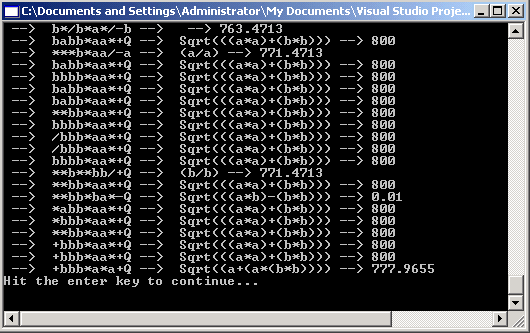Figure 4 - Generation 200 with a perfect fitness

The measurements of our right triangles that we used are shown below: (note our ruler goes out to the 6th decimal place, so one would need an electron microscope to measure accurate hypotenuses )

float[,] measure = new float[8,3]{{3,4,5}, {1, 1, 1.4142f}, {1, 2, 2.23607f}, {3, 1, 3.16228f}, {6, 13, 14.31782f}, {4,3,5}, {1,3, 3.16228f}, {2, 1, 2.23607f}};

Note that we don't always converge on the correct solution with the parameters and measurements  that we are using.  Below is another convergence.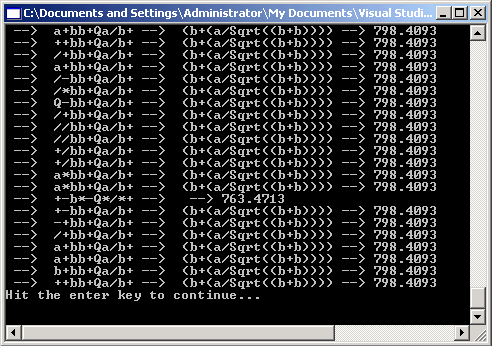Figure 5- Convergence with a lower fitness

This solution reads as b + (a/Sqrt(2b)), which is not the correct solution, but seems to produce a relatively small error on the measurements that we have chosen.

Conclusion

GEP's are fun to experiment with.  If you are really up for a challenge, you can try to produce the quadratic equation shown in the symbol tree at the top of this article based on sample solutions with known a, b, and c measurements against known roots.  You can also experiment with GEP on logic expressions such as Morgan's law ( ~(a | b) = (~a)  & (~b).  Anyway, enjoy playing with this cool algorithm as we delve deeper into the world of AI in C# and  .NET.

References

Gene Expression Programming In Problem Solving, Candida Ferreira
Postfix Evaluation, Premshree Pillai

Recommended Free Ebook
Similar Articles